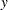COORDINATES Statement
COORDINATES coordinate-variables ;

The following two options give the names of the variables in the DATA= data set that contains the values of theandcoordinates of the data.

Only one COORDINATES statement is allowed, and it is applied to all the analysis variables. In other words, it is assumed that all the VAR variables have the sameandcoordinates.

XCOORD=(variable-name)
XC=(variable-name)
X=(variable-name)

gives the name of the variable that contains thecoordinate of the data in the DATA= data set.

YCOORD=(variable-name)
YC=(variable-name)
Y=(variable-name)

gives the name of the variable that contains thecoordinate of the data in the DATA= data set.